### What are Shapes?

In geometry, a shape can be defined as the form of an object or its outline, outer boundary or outer surface.

Everything we see in the world around us has a shape. We can find different basic shapes such as the two-dimensional square, rectangle, and oval or the three-dimensional rectangular prism, cylinder, and sphere in the objects we see around us. These geometric shapes appear in objects we see all around us such as credit cards, bills and coins, rings, photo frames, dart boards, houses, windows, tall buildings, flower pots, toy trains, and balloons.

### Different Types of Shapes

Shapes can be classified into open and closed shapes.Closed geometric shapes can further be put into two broad categories, namely two-dimensional and three-dimensional shapes.

2-Dimensional [2-D] Circle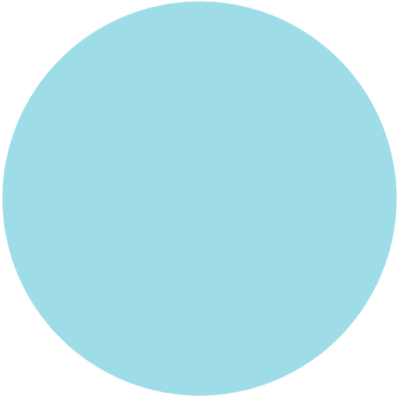3-Dimensional [3-D]
CubeToday we are going to focus on 2-Dimensional shapes. Let’s learn a bit more about some shapes.### Square:

A shape that has four equal sides and four equal angles.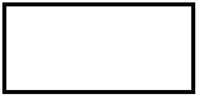### Rectangle:

A four-sided shape that is made up of two pairs of parallel lines and that has four right angles; especially : a shape in which one pair of lines is longer than the other pair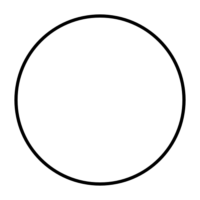### Circle:

A perfectly round shape – a line that is curved so that its ends meet and every point on the line is the same distance from the center.### Ellipse:

A curved shape that is made by an unbroken line. An ellipse is shaped like an egg, but with equal ends. It is a perfect oval.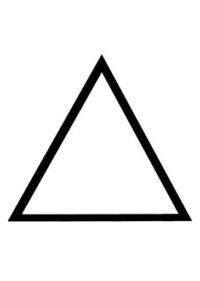### Equilateral Triangle:

A flat figure with three equal sides and three equal angles.### Isosceles Triangle:

A triangle that has only two sides of equal length and two equal angles.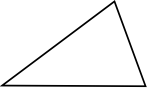### Scalene Triangle:

A triangle that has three unequal sides and three unequal angles.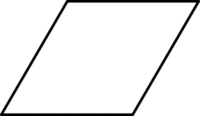### Rhombus:

A flat figure that has four equal sides and usually no right angles.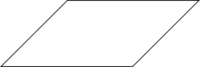### Parallelogram:

A flat figure having four sides, with opposite sides parallel and of equal length.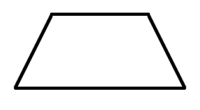### Trapezoid:

A flat figure with four sides; two of the sides are parallel and two are not.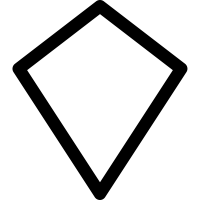### Kite:

A four sided flat shape with straight sides that has two pairs of sides and each pair is made of two adjacent sides (they meet) that are equal in length.### Pentagon:

A flat figure having five sides and five angles.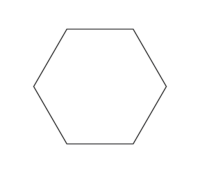### Hexagon:

A flat figure having six sides and six angles.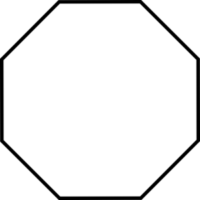### Octagon:

A flat figure with eight sides and eight angles.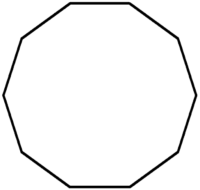### Decagon:

A flat figure with ten sides and ten angles.

In our STEM Club session on November 4th we will be learning about even more shapes! We will then use geometric shapes to make unique works of art! If you would like to participate all you need is some paper, scissors, glue, and to print off a few copies of this sheet of shapes.